Courses

# Test: Arithmetic - 1 (2019-2019)

## 20 Questions MCQ Test UPSC Topic Wise Previous Year Questions | Test: Arithmetic - 1 (2019-2019)

Description
This mock test of Test: Arithmetic - 1 (2019-2019) for UPSC helps you for every UPSC entrance exam. This contains 20 Multiple Choice Questions for UPSC Test: Arithmetic - 1 (2019-2019) (mcq) to study with solutions a complete question bank. The solved questions answers in this Test: Arithmetic - 1 (2019-2019) quiz give you a good mix of easy questions and tough questions. UPSC students definitely take this Test: Arithmetic - 1 (2019-2019) exercise for a better result in the exam. You can find other Test: Arithmetic - 1 (2019-2019) extra questions, long questions & short questions for UPSC on EduRev as well by searching above.
QUESTION: 1

### The average marks of 100 students are given to be 40. It was found later that marks of one student were 53 which were misread as 83. The corrected mean marks are 

Solution:

Total marks of 100 students
= 40 × 100 = 4000
correct mean marks of 100 students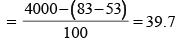QUESTION: 2

### Rakesh had money to buy 8 mobile handsets of a specific company. But the retailer offered very good discount on that particular handset. Rakesh could buy 10 mobile handsets with the amount he had. What was the discount the retailer offered? 

Solution:

Let price of each mobile is ₹ 1000.
Then, Price of 8 mobile = ₹ 8000.
Price of 10 mobile = ₹ 10000.
Percentage discount =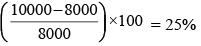QUESTION: 3

### Ena was born 4 years after her parents, marriage. Her mother is three years younger than her father and 24 years older than Ena, who is 13 years old. At what age did Ena’s father get married? 

Solution:

Mother is 24 years older then Ena.
So, at the time of Ena's birth, mother's age was 24 years.
Again, Ena was born after 4 years of their parents wedding
So, mother's age at the time of wedding = 24 – 4 = 20 years
Her father is 3 years older than her mother
So, her father's age at the time of their wedding = 20 + 3 = 23 years.

QUESTION: 4

If x is greater than or equal to 25 and y is less than or equal to 40, then which one of the following is always correct?



Solution:

ATQ,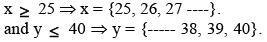For (y – x) maximum, y should be maximum and x should be minimum.
(y – x) ≤ (40 – 25)
∴ (y – x) ≤ 15.
Hence, (y – x) is away less then or equal to 15.

QUESTION: 5

In an examination, A has scored 20 marks more than B. If B has scored 5% less marks than A, how much has B scored?



Solution:

Let B's score is n and, A's score is (m)
ATQ,  m – n = 20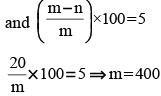B's score = 400 – 20 = 380

QUESTION: 6

The ratio of a two-digit natural number to a number formed by reversing its digits is 4 : 7.The number of such pairs is



Solution:

Let the two-digit natural number are 10x + y on reversing its digits, number formed 10y + x
ATQ,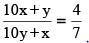7(10x + y) = 4(10y + x)
70x + 7y = 40y + 4x
66x = 33y
2x = y
Hence, such number are (12, 24, 36, 48)

QUESTION: 7

All members of a club went to Mumbai and stayed in a hotel. On the first day, 80% went for shopping and 50% went for sightseeing, whereas 10% took rest in the hotel. Which of the following conclusion(s) can be drawn from the above data?
1. 40% members went for shopping as well as sightseeing.
2. 20% members went for only shopping.
Select the correct answer using the code given below :



Solution:

Percentage of members of the club went for shopping or Sighteeing 100 – 10 = 90%
1.  Percent of members went for both = 80 + 50 – 90 = 40%
2.  Percent of members went for only shopping = 80 – 40 = 40%
Hence, only statement 1 is true.

QUESTION: 8

In a conference, out of a total 100 participants, 70 are Indians. If 60 of the total participants are vegetarian, then which of the following statements is/are correct?
1. At least 30 Indian participants are vegetarian.
2. At least 10 Indian participants are non-vegetarian.
Select the correct answer using the codes given below :



Solution:

Number of non-Indian participants = 100 – 70 = 30.
1. Number of vegetarian = 60.
Number of Indian that are vegetarian ≥ (60 – 30) ≥ 30
2. Number of non-vegetarian
= 100 – 60.
= 40.
Maximum Number of non-Indian that are non-vegetarian = 40 – 30 = 10
Hence, at least 10 Indian are non-vegetarian.

QUESTION: 9

Rakesh and Rajesh together bought 10 balls and 10 rackets. Rakesh spent ₹1300 and Rajesh spent ₹1500. If each racket costs three times a ball does, then what is the price of a racket?



Solution:

Cost of 10 balls + 10 rackets = 1300 + 1500 = 2800
∴ Cost of 1 ball + 1 racket = 280 ...(i)
ATQ,  Cost of 1 racket = 3 × Cost of 1 ball
∴ From (i)
Cost of 4 balls = 280
Cost of 1 ball   = 70
∴ Cost of 1 racket  = ₹ 210

QUESTION: 10

In 2002, Meenu’s age was one-third of the age of Meera, whereas in 2010, Meenu’s age was half the age of Meera. What is Meenu’s year of birth?



Solution:

Let Meera age in 2002 = x years
and Meenu age in 2002 = y years
∴ x = 3y and x + 8 = 2(y + 8)
⇒ 3y + 8 = 2y + 16 ⇒ y = 8
Meenu's age = 8 years
Hence, Meenu's year of birth = 2002 – 8 = 1994

QUESTION: 11

Raju has ₹ 9000 with him and he wants to buy a mobile handset; but he finds that he has only 75% of the amount required to buy the handset. Therefore, he borrows ₹ 2000 from a friend. Then



Solution:

Price of the mobile phone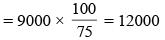After taking ₹ 2000 from his friend, Raju has 9000 + 2000 = ₹ 11000.
Thus, he still does not have enough amount to buy the handset.

QUESTION: 12

In a school every student is assigned a unique identification number. A student is a football player if and only if the identification number is divisible by 4, whereas a student is a cricketer if and only if the identification number is divisible by 6. If every number from 1 to 100 is assigned to a student, then how many of them play cricket as well as football?



Solution:

L.C.M. of 4 and 6 = 12.
Hence the number assign to the students who play cricket as well as football is divisible by 12.
Number divisible by 12 are 12, 24, 36......
Total number of such Numbers up to 100 = 8. and that are 12, 24, 36, 48, 60, 72, 84, 96.
Hence required number = 8

QUESTION: 13

A and B are two heavy steel blocks. If B is placed on the top of A, the weight increases by 60%. How much weight will reduce with respect to the total weight of A and B, if B is removed from the top of A?



Solution:

Let weight of block A = x kg
and weight of block B = y kg
total weight of two blocks = (x + y) kg
ATQ,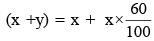x + y = 1.6x ⇒  y = 0.6x.
Now, when block B is removed, then percent reduction in the weight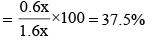QUESTION: 14

The number of times the digit 5 will appear while writing the integers from 1 to 1000 is



Solution:

Number of times ‘5’ appear from 1 to 100 = 20
Number of times ‘5’ appear from 101 to 200 = 20
Similary, number of times ‘5’ appear from 201 to 499 = 60
Number of times '5' appear from ‘600 to 1000’ = 80
Number of times ‘5’ appear from ‘500 to 599’. = 120
Total number of times ‘5’ appear from 1 to 1000
= 20 + 20 + 60 + 80 + 120 = 300

QUESTION: 15

Number 136 is added to 5B7 and the sum obtained is 7A3, where A and B are integers. It is given that 7A3 is exactly divisible by 3.
The only possible value of B is



Solution:

'7 A3' is divisible by 3 only when sum of its digits be divisible by 3.
7 + A + 3 = 10 + A
For, A = 2, 5 and 8. (10 + A) would be divisible by 3.
ATQ,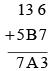for A = 2, B = 8,
136 + 587 = 723
Hence, B = 8

QUESTION: 16

A family has two children along with their parents. The average of the weights of the children and their mother is 50 kg. The average of the weights of the children and their father is 52 kg. If the weight of the father is 60 kg, then what is the weight of the mother?



Solution:

Let the sum of the weight of two childrens is x kg. and weight of mother and father are y and z kg respectively.
ATQ,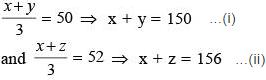here, z = 60, (given)
from (ii)
x + 60 = 156
x = 96
From (i),
96 + y = 150
y = 150 – 96 = 54 kg.

QUESTION: 17

In a group of 15 people; 7 can read French, 8 can read English while 3 of them can read neither of these two languages. The number of people who can read exactly one language is



Solution:

Number of people who read either or both languages.
= 15 – 3 = 12.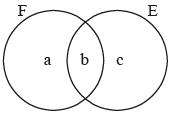∴ a + b + c = 12
Number of people who read French,
a + b = 7
Number of people read English,
b + c = 8
∴ (a + b) + (b + c) = 7 + 8 = 15
⇒ (a + b + c) + b = 15
⇒ 12 + b = 15 Þ b = 3
∴ a = 4 and c = 5
Hence, number of people who can read exactly one language
=  a + c = 9

QUESTION: 18

A wall clock moves 10 minutes fast in every 24 hours. The clock was set right to show the correct time at 8:00 a.m. on Monday. When the clock shows the time 6:00 p.m. on Wednesday, what is the correct time?



Solution:

In each hour clock moves fast by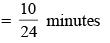Number of hours between 8:00 am on monday and 6:00 pm on wednesday
= 58 hours.
So, clock will move fast in  58 hours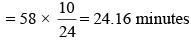So, current time
= 6 – 24.16 = 5:36 pm.

QUESTION: 19

An 8-digit number 4252746B leaves remainder 0 when divided by 3. How many values of B are possible?



Solution:

A number is divisible by '3' if sum of its digits be divisible by 3.
Here, Given number is 4252746 B
Sum of its digits = 4 + 2 + 5 + 2 + 7 + 4 + 6 + B
= 30 + B.
For, B = 0, 3, 6, 9;
Sum of digits will be divisible by '3'.
Hence, number of values of  B = 4.

QUESTION: 20

Sunita cuts a sheet of paper into three pieces. Length of first piece is equal to the average of the three single digit odd prime numbers. Length of the second piece is equal to that of the first plus one-third the length of the third. The third piece is as long as the other two pieces together. The length of the original sheet of paper is



Solution:

Let first Piece, second Pieec and third Piece are x, y and z respectively.
Now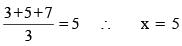According to question,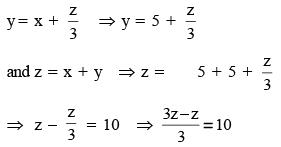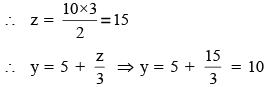∴ The length of the original sheet
= x + y + z
= 5 + 10 + 15
= 30 units